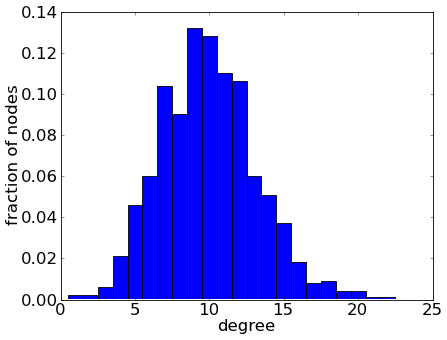# Math Insight

### One of the simplest types of networks

#### A task with incomplete information

Imagine that I asked you to generate a undirected network containing 1000 nodes and about 1% of the possible edges. Clearly those instructions aren't enough to determine exactly what type of network I wanted, as I gave you so little information. But, imagine that you weren't able to have any further communication with me and so needed to generate the graph without asking me any clarifying questions.

In coming up with a method to generated the graph, your thought process might go something like this. Since you weren't told anything to the contrary, you might as well assume that all nodes and edges are identical. It's also reasonable to not allow connections from a node onto itself (nor multiple connections between pairs of nodes). This means that there are a total of $$\binom{1000}{2} = 1000 \cdot 999/2 = 499,500$$ possible edges in the graph. To specify the network according your instruaction, you just need to choose which 1% of the edges to include in the network, i.e., you must choose 4,995 edges.

Since you don't know which 1% of the connections to include in the network, you might as well choose them randomly. First, you write a computer program that approximately generates a random variable $X$ so that is $X=1$ with 1% probability and $X=0$ otherwise. Then, you write a computer program that goes through each of the possible 499,500 edges, generates an instance of $X$ for each. For example, for the edge between node $i$ and $j$, you might call the corresponding random variable $X_{ij}$. The computer then selects the edge between node $i$ and $j$ for inclusion into the network if $X_{ij}=1$; if $X_{ij}=0$, the edge is not included. When you run the program, you get a network with 4965 edges, which is about 0.994% of the possible edges. You deem this close enough to 1% and decide to use the result for your network.

#### The resulting network

What type network did you generate with your computer program? You may not have thought about it, but you made one important (and natural) assumption when you generated the graph. When you generated the 499,500 random $X$'s, the computer program did not let the resulting value of one variable, say $X_{ij}$, influence the probability of another variable, say $X_{kl}$ (for different nodes $k$ and $l$). No matter what values were selected for the other $X$'s, each $X_{ij}$ was selected to be 1 with probability 0.01. In other words, the computer program generated each of the $X$'s independently.

This approach wasn't your only option. You could have introduced dependencies among the random variables. For example, you could have made the probability that $X_{ij}=1$ either increase or decrease depending on how many connections the nodes $i$ and $j$ already have (compensating in some way to keep the total number of connections you end up with around 1% of the possible connections). Or, you could have avoided using random variables altogether and, after numbering the nodes from 1 to 1000, connected each node with the 10 nodes with the closest numbers. If you had a lot of time to spend on this task, I'm sure you could have come up some intricate scheme to give the network very complicated structure.

The network you generated was an example of an Erdös-Rényi random network1 (or ER random network), the simplest type of random network. Since all edges are independent, ER random networks are the natural type of network to assume when thinking of random networks. For this reason, people often refer to ER random networks as simply “random networks,” and may view deviations from the Erdös-Rényi model as “non-randomness.“ But the edges in a graph can still be quite random even if their are correlations among them (though such graphs have less entropy, as can be inferred from the box, above).

One important consequence of generating the edges independently is that the degree distribution of the network is fairly tight. You can think of the independence among edges as meaning the edges couldn't communicate with one another when they were being generated. When the network was generated, the edges weren't allowed to conspire and agree to concentrate around a few nodes and turn them into hubs. Instead, independent edges end up spreading themselves fairly evenly across the nodes, and the degree distribution is a binomimal distribution. The result is that, in the network you generated, most neurons had a degree that was fairly close to the average degree of 10, as shown below.